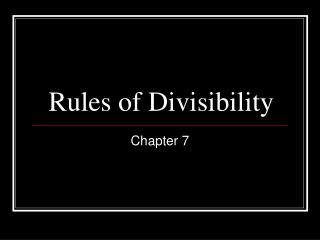DownloadDownload PresentationRules of Divisibility

# Rules of Divisibility

Télécharger la présentation## Rules of Divisibility

- - - - - - - - - - - - - - - - - - - - - - - - - - - E N D - - - - - - - - - - - - - - - - - - - - - - - - - - -
##### Presentation Transcript

1. Rules of Divisibility Chapter 7

2. Rule of “2” • If the number ends with:0, 2, 4, 6, or 8 • It is divisible by 2 • Divisible=Divide • Divisible means a number can go into another number evenly with no remainder

3. The Rule of “2” • Example: • 48, 56, 22, 10 and 34 • Are all divisible by 2 • Explain why: They all end with a number that is divisible by 2

4. Practice • 88, 95, 108, 2004, 26, 44 • Which number is not divisible by “2” • Answer 95 • Explain why? • 5 is not divisible by 2

5. Rule of “5” • Rule of “5” • The number must end with 5 or 0 • Example: 550 • Ends with 0, so it is divisible by 5

6. Practice • Which numbers are divisible by 5 ? • 262, 265, 360, 455 and 291 • Answer: • 265 • 360 • 455 • Explain why? • The numbers end 5 or 0

7. Rule of “10” • Rule of 10 • The number has to end with 0 to be divisible by 10 • Example: 690 • Ends with 0, so 690 is divisible by 10

8. Practice • Which number is divisible by 10 • 550 or 600 • Answer • Both 550 and 600 • Explain why: They both end with 0

9. Rule of “4” • Rule of “4” • The last two digits of a number must be divisible by 4 • Example: 444 • Take the last two digits of the number 444 • Divide by 4: 44÷4= 11 • 444 is divisible by 4

10. Practice • Which number is divisible by 4 • 460 or 328 • Answer • Both • Why? • 4 can go into 28 evenly • 4 can go in 60 evenly

11. Rule of “3” • The sum of the digits must be divisible by 3 • Example: 240 • Add up each digit: 2 + 4 + 0 = 6 • Now divide 6÷3=2 • 3 goes into 6 with no remainder so 240 is divisible by 3

12. Practice • Which number is divisivble by 3 • 24 or 81 • Answer • Both • Why? • 2 + 4=6, three goes into six evenly • 8 + 1= 9 three goes into nine evenly

13. Rule of 9 • The sum of the digits must be divisible by 9 • Example: 360 • 3 + 6 + 0 = 9 • Now take the sum which is “9” and divide • 9 ÷ 9 = 1 • 360 is divisible by 9

14. Practice • Which number is divisible by 9? • 540 or 361 • Answer • 540 • Why 5 + 4 + 0 = 9 • 9 goes into 9 evenly with no remainder